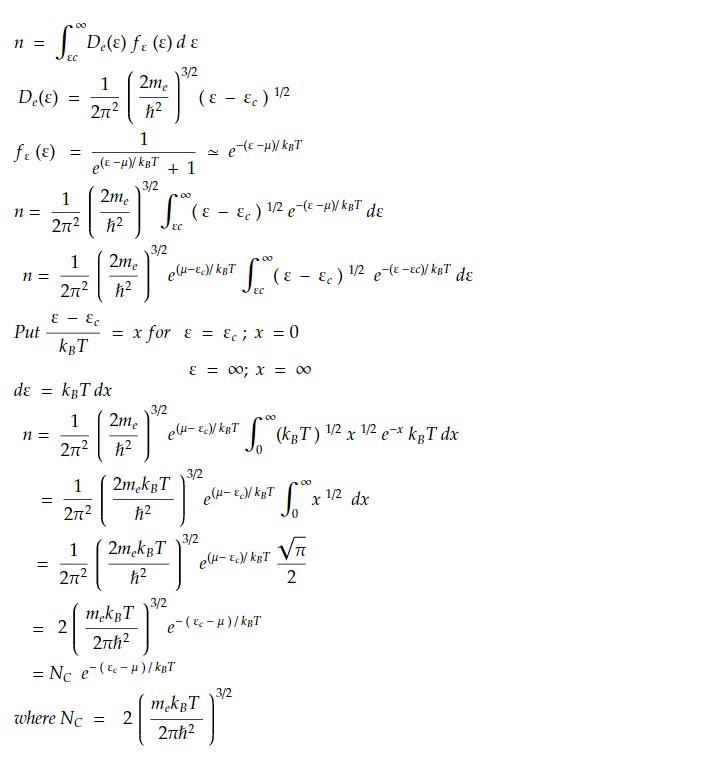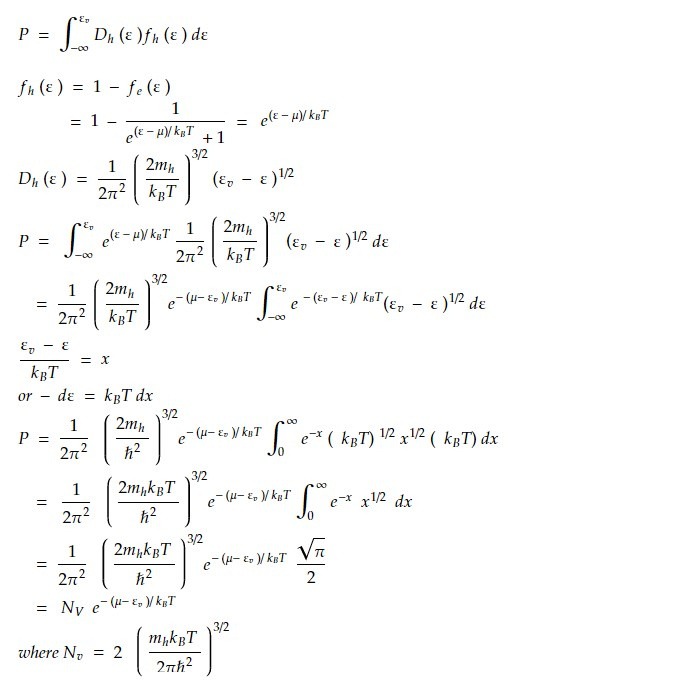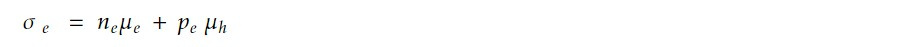# Expression for concentration of carrier in an intrinsic carrier in semi conductor.

In an intrinsic semiconductor, the concentration of carriers (electrons and holes) is determined by the intrinsic carrier concentration, denoted as ni. The intrinsic carrier concentration is a function of temperature and is given by:

ni² = Nv . Nc . exp(-Eg/kT)

where Nv is the effective density of states in the valence band, Nc is the effective density of states in the conduction band, Eg is the energy gap between the valence and conduction bands, k is the Boltzmann constant, and T is the temperature in Kelvin.

At room temperature (around 300 K), the intrinsic carrier concentration for silicon is approximately 1.5 x 10^10 carriers/cm^3. This value represents the concentration of electrons and holes that are thermally generated in the semiconductor due to thermal energy. In an intrinsic semiconductor, the concentration of electrons and holes is equal, and the material is electrically neutral overall.

When impurities are introduced into the semiconductor material to create doped regions, the concentration of carriers can be greatly increased. In n-type doping, for example, the concentration of electrons is increased by introducing impurities such as phosphorus, which have extra electrons that can become mobile carriers. Similarly, in p-type doping, the concentration of holes is increased by introducing impurities such as boron, which have fewer electrons and thus create holes in the valence band.

In summary, the concentration of carriers in an intrinsic semiconductor is determined by the intrinsic carrier concentration, which is a function of temperature and the material properties. The concentration of carriers can be increased by doping the semiconductor material with impurities to create regions with excess electrons or holes.

Derivation of concentration of carrier in an intrinsic carrier in semi conductor

Under condition of thermal equilibrium, concentration of electron isSimilarly condition of thermal equilibrium of holes in valence band isFrom law of mass ni² = npεg is band gap energy

Now, electrical conductivity of intrinsic semi conductor due to electron and holes areAlso drift current density due to eComparing equation 1, 2 and 3Intrinsic semiconductor at thermal equilibrium is

n = p = ni𝜎o is constant independent of temperature.

This note is a part of the Physics Repository.